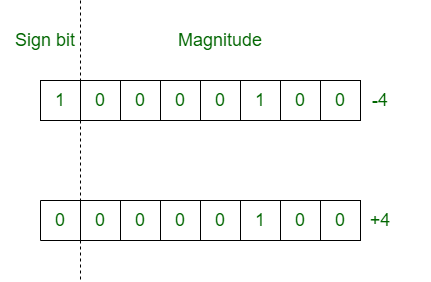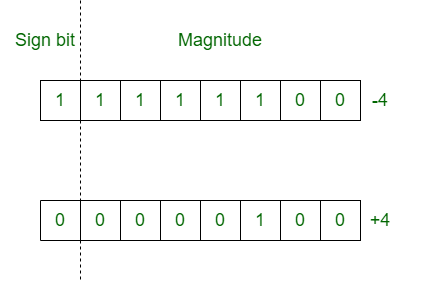Related Articles
Difference between Signed magnitude and 2’s complement
• Last Updated : 03 Jul, 2020

1. Signed Magnitude Method :
In the signed magnitude method number is divided into two parts: Sign bit and magnitude. Sign bit is 1 for negative number and 0 for positive number. Magnitude of number is represented with the binary form of the number.
Example: Lets take 8 bits register.2. 2’s Complement Method :
In 2’s complement method, positive numbers are represented in the same way as they are represented in sign magnitude method. But if the number is negative, first represent the number with positive sign and then take 2’s complement of that number.
Example: Lets take 8 bits register.Difference between Signed Magnitude and 2’s Complement Method :

SIGNED MAGNITUDE METHOD2’s COMPLEMENT METHOD
It is a method to denote fixed point signed numbers.It is also used to denote fixed point signed numbers.
Number is divided into two parts.Number is considered as a whole.
Sign bit is considered explicitly.Sign bit is not considered explicitly.
Additional hardware is required for resultant sign of arithmetic.No additional hardware is required in 2’s complement method.
Addition and subtraction are performed on separate hardware.Addition and subtraction are performed by using adder only.
It has two different representation for 0. One is +0 and second is -0. (+0 : 0000 0000) & (-0 : 1000 0000)0 has only one representation for -0 and +0 (+0 or -0 : 0000 0000).
It is non-weighted system.It assigns negative weight to the sign bit.

Attention reader! Don’t stop learning now. Get hold of all the important CS Theory concepts for SDE interviews with the CS Theory Course at a student-friendly price and become industry ready.

My Personal Notes arrow_drop_up